# For each of the following statements state whether true(T) or false(F).Question:

For each of the following statements state whether true(T) or false(F).
(i) Two circles with different radii are similar.
(ii) Any two rectangles are similar.
(iii) If two traingles are similar, the their corresponding angles are equal and their corresponding sides are equal.
(iv) The length of the line segment joining the mid points of any two sides of a trinagle is equal to the half the length of the third side.
(v) In △ABC, AB = 6 cm, ∠A = 450 and AC = 8 cm and in △DEF, DF = 9 cm, ∠D = 450 and DE = 12 cm, then △ABC ∼ △DEF.
(vi) The polygon formed by joining the mid points of the sides of a quadrilateral is a rhombus.
(vii) The ratio of areas of two similar triangles is equal to the ratio of their corresponding angle-bisector segments.
(viii) The ratio of the perimeters of two similar triangles is same as the ratio of their corresponding medians.
(ix) If O is any point inside a rectangle ABCD then OA2 + OC2 = OB2 + OD2
(x) The sum of the squares on the sides of a rhombus is equal to the sum of the squares on its diagonals.

Solution:

(ii) True
Two circles of any radii are similar to each other.

(i) False
Two rectangles are similar if their corresponding sides are proportional.

(iii) Falase
If two traingles are similar, the their corresponding angles are equal and their corresponding sides are proportional.

(iv) True
Suppose ABC is a triangle and M, N are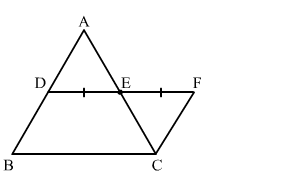Construction: DE is expanded to F sich that EF = DE

To Prove $=\mathrm{DE}=\frac{1}{2} \mathrm{BC}$

AE = EC        (E is the mid point of AC)
DE = EF        (By construction)
AED = CEF      (Vertically Opposite angle)
By SAS criterion, △ADE ≅ △CEF
⇒ BD = CF
Since, ∠ADE and ∠EFC are alternate angle
Hence, AD ∥ CF and BD ∥ CF
When two sides of a quadrilateral are parallel, then it is a parallelogram
∵DF = BC and BD ∥ CF
∴BDFC is a parallelogram
Hence, DF = BC
⇒ DE + EF = BC

$\Rightarrow \mathrm{DE}=\frac{1}{2} \mathrm{BC}$

(v) False
In △ABC, AB = 6 cm, ∠A = 45 and AC = 8 cm and in △DEF, DF = 9 cm, ∠D = 45 and DE = 12 cm, then △ABC ∼ △DEF.

In △ABC and △DEF
∠A = ∠D = 45

$\frac{\mathrm{AB}}{\mathrm{AC}} \neq \frac{\mathrm{DE}}{\mathrm{DF}}$

So △ABC is not similar to △DEF

(vi) False
The polygon formed by joining the mid points of the sides of a quadrilateral is a parallelogram.

(vii) True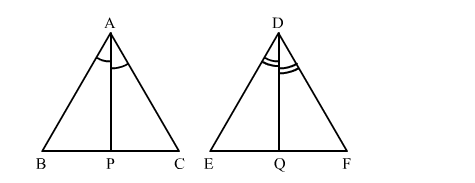Given: △ABC ∼ △DEF

To Prove $=\frac{\operatorname{Ar}(\triangle \mathrm{ABC})}{\mathrm{Ar}(\triangle \mathrm{DEF})}=\left(\frac{\mathrm{AP}}{\mathrm{DQ}}\right)^{2}$

Proof: In △ABP and △DEQ
∠BAP = ∠EDQ        (As ∠A = ∠D, so their Half is also equal)
∠B = ∠E                   (△ABC ∼ △DEF)
By AA criterion, △ABP ∼ △DEQ

$\frac{\mathrm{AB}}{\mathrm{DE}}=\frac{\mathrm{AP}}{\mathrm{DQ}}$   ...........(i)

Since, △ABC ∼ △DEF

$\therefore \frac{\mathrm{Ar}(\triangle \mathrm{ABC})}{\mathrm{Ar}(\triangle \mathrm{DEF})}=\left(\frac{\mathrm{AB}}{\mathrm{DE}}\right)^{2}$

$\Rightarrow \frac{\operatorname{Ar}(\triangle \mathrm{ABC})}{\operatorname{Ar}(\triangle \mathrm{DEF})}=\left(\frac{\mathrm{AP}}{\mathrm{DQ}}\right)^{2} \quad[\mathrm{Using}(1)]$

(viii) True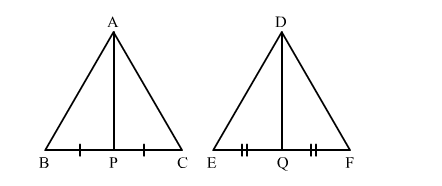Given: △ABC ∼ △DEF

To Prove $=\frac{\text { Perimeter }(\triangle \mathrm{ABC})}{\text { Perimeter }(\triangle \mathrm{DEF})}=\frac{\mathrm{AP}}{\mathrm{DQ}}$

Proof: In △ABP and △DEQ
∠B = ∠E                  (∵△ABC ∼ △DEF)
∵△ABC ∼ △DEF

$\therefore \frac{\mathrm{AB}}{\mathrm{DE}}=\frac{\mathrm{BC}}{\mathrm{EF}}$

$\Rightarrow \frac{\mathrm{AB}}{\mathrm{DE}}=\frac{2 \mathrm{BP}}{2 \mathrm{EQ}}$

$\Rightarrow \frac{\mathrm{AB}}{\mathrm{DE}}=\frac{\mathrm{BP}}{\mathrm{EQ}}$

By SAS criterion, △ABP ∼ △DEQ

$\frac{\mathrm{AB}}{\mathrm{DE}}=\frac{\mathrm{AP}}{\mathrm{DQ}}$      .....(1)

Since, △ABC ∼ △DEF

$\therefore \frac{\text { Perimeter }(\triangle \mathrm{ABC})}{\text { Perimeter }(\triangle \mathrm{DEF})}=\frac{\mathrm{AB}}{\mathrm{DE}}$

$\Rightarrow \frac{\text { Perimeter }(\triangle \mathrm{ABC})}{\text { Perimeter }(\triangle \mathrm{DEF})}=\frac{\mathrm{AP}}{\mathrm{DQ}} \quad[\mathrm{U} \sin g(1)]$

(ix) True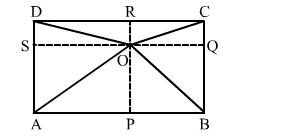Suppose ABCD is a rectangle with O is any point inside it.
Construction: Join OA, OB, OC, OD and draw two parallel lines SQ ∥ AB ∥ DC and PR ∥ BC ∥ AD
To prove: OA2 + OC2 = OB2 + OD2
Proof:
OA2 + OC2 = (AS2 + OS2) + (OQ2 + QC2)             [Using Pythagoras theorem in right triangle AOP and COQ]
= (BQ2 + OS2) + (OQ2 + DS2
= (BQ2 + OQ2) + (OS2 + DS2)                                 [Using Pythagoras theorem in right triangle BOQ and DOS]
= OB2 + OD2
Hence, LHS = RHS

(x) True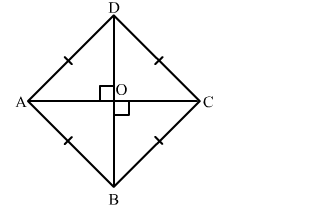Suppose ABCD is a rhombus having AC and BD its diagonals.
Since, the diagonals of a rhombus perpendicular bisect each other.
Hence, AOC is a right angle triangle
In right triangle AOC
By using Pythagoras theorem, we have

$\mathrm{AB}^{2}=\mathrm{AO}^{2}+\mathrm{OB}^{2}$

$\Rightarrow \mathrm{AB}^{2}=\left(\frac{\mathrm{AC}}{2}\right)^{2}+\left(\frac{\mathrm{BD}}{2}\right)^{2} \quad[\because$ Diagonals of a rhombus perpendicularly bisect each other $]$

$\Rightarrow \mathrm{AB}^{2}=\frac{\mathrm{AC}^{2}}{4}+\frac{\mathrm{BD}^{2}}{4}$

$\Rightarrow 4 \mathrm{AB}^{2}=\mathrm{AC}^{2}+\mathrm{BD}^{2}$

$\Rightarrow \mathrm{AB}^{2}+\mathrm{AB}^{2}+\mathrm{AB}^{2}+\mathrm{AB}^{2}=\mathrm{AC}^{2}+\mathrm{BD}^{2}$

$\Rightarrow \mathrm{AB}^{2}+\mathrm{BC}^{2}+\mathrm{CD}^{2}+\mathrm{DA}^{2}=\mathrm{AC}^{2}+\mathrm{BD}^{2} \quad[\because$ All sides of a rhombus are equal $]$